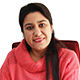×The Free 30-Day CAT RC Course

"It is designed to help you excel in the upcoming CAT 2023 exam"

-By Lavleen Kaur Kapoor. Over 1,73,000 Subscribers

No thanks >

OPEN

## IBSAT, CAT, XAT, NMAT, GMAT

OPEN#### Get Personalized MBA Mentorship

Connect with my team on WhatsApp for unbiased MBA College Selection advice.

# Preparation Material: Mathematical Skills - H.C.F and L.C.M

MAT Exam Date(PBT) : 21 MAY 2023 (Sunday)MBA aspirants who are appearing in MAT May 2023 may prepare for Mathematical skills section with questions on HCF & LCM :

Question 1) The greatest number which on dividing 1657 and 2037 leaves remainders 6 and 5 respectively, is:

A. 123

B. 127

C. 235

D. 305

Soultion:
Required number = H.C.F of (1657-6) and (2037-5)
= H.C.F of 1651 - 2032 = 127.

Question 2) Which of the following has the most number of divisors?

A. 99

B. 101

C. 176

D. 182

Soultion:
99 = 1 x 3 x 3 x 11
101 = 1 x  101
176 = 1 x 2 x 2 x 2 x 2 x 11
182 = 1 x 2 x 7 x 13

So, divisiors of 99 are 1, 3, 9, 11, 33, 99
Divisiors of 101 are 1 and 101
Divisiors of 176 are 1, 2, 4, 8, 11, 16, 22, 44, 88, and 176
Divisors of 182 are 1, 2, 7, 13, 14, 26, 91 and 182.

Hence, 176 has the most number of divisors.

Question 3) The H.C.F. of 9/10, 12/15, 18/35 and 21/40 is:

A. 3/5

B. 252/5

C. 3/1400

D. 63/700

Solution:
Required  H.C.F. = H.C.F. of 9, 12, 18, 21 / L.C.CM. of 10, 15, 35, 40 = 3/1400

Question 4) If the sum of two numbers is 55 and the H.C.F. and L.C.M. of these numbers are 5 and 120 respectively, then the sum of the reciprocals of the numbers is equal to:

A. 55/601

B. 601/55

C. 11/120

D. 120/11

Solution:
Let the numbers be a and b.
Then, a+b=55 and ab = 600
Therefore, the required sum = 1/a + 1/b = a+b / ab = 55/600 = 11/120

Question 5) The L.C.M of two numbers is 48. The numbers are in the ratio 2:3. Then sum of the number is:

A. 28

B. 32

C. 40

D. 64

Solution:
Let the numbers be 2x and 3x.
Then, their L.C.M = 6x.
So, 6x = 48 or x=8.
Therefore, The numbers are 16 and 21.
Hence, required sum = (16+24) = 40.